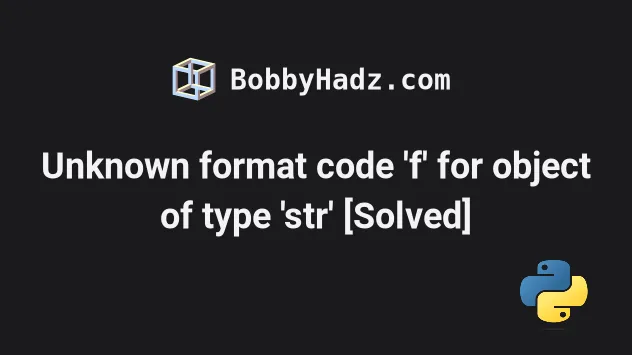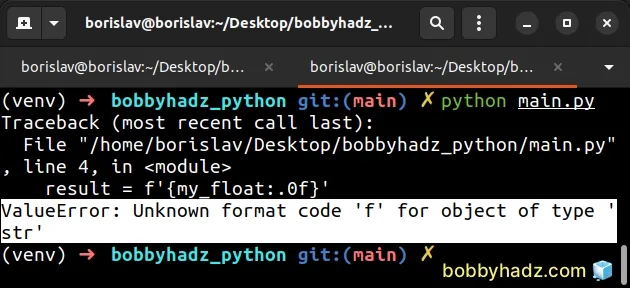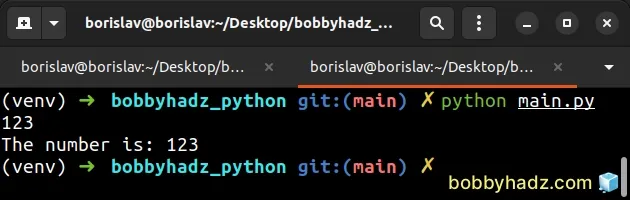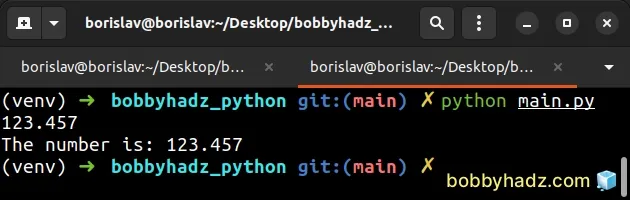# Unknown format code 'f' for object of type 'str' [Solved]Last updated: Jun 23, 2023
2 min## #Unknown format code 'f' for object of type 'str' [Solved]

The Python "ValueError: Unknown format code 'f' for object of type 'str' " occurs when you try to use the `f` format code with a value that is a string.

To solve the error, use the `float()` class to convert the string to a floating-point number.

Here is an example of how the error occurs.

main.py
```Copied!```my_float = '123.456789'

# ⛔️ ValueError: Unknown format code 'f' for object of type 'str'
result = f'{my_float:.0f}'
``````We used a formatted string literal.

Formatted string literals (f-strings) let us include expressions inside of a string by prefixing the string with `f`.

The expressions have to be wrapped in curly braces - `{expression}`.

Formatted string literals also enable us to use the format-specific mini-language in expression blocks.

The `f` format code stands for fixed-point notation.

The error is caused because the `my_float` variable stores a string instead of a floating-point number.

To resolve the issue, use the float class to convert the value to a floating-point number.

main.py
```Copied!```my_float = '123.456789'

result = f'{float(my_float):.0f}'
print(result)  # 👉️ 123

result = f'The number is: {float(my_float):.0f}'
print(result)  # 👉️ The number is: 123
``````The `float` class converts the supplied value to a floating-point number.

As shown in this section of the docs, the digit after the period is the number of decimal places the number should have.

main.py
```Copied!```my_float = '123.456789'

result = f'{float(my_float):.3f}'
print(result)  # 👉️ 123.457

result = f'The number is: {float(my_float):.3f}'
print(result)  # 👉️ The number is: 123.457
``````The `f` character in the expression stands for fixed-point notation.

It is used to format the number as a decimal with exactly N digits following the decimal point.

## #Solving the error when using `str.format()`

You might also get the error when using the `str.format()` method.

main.py
```Copied!```my_float = '123.456789123'

# ⛔️ ValueError: Unknown format code 'f' for object of type 'str'
result = '{:.0f}'.format(my_float)
``````

The solution to the error is the same - you have to convert the string to a floating-point number when passing it to str.format.

main.py
```Copied!```my_float = '123.456789123'

result = '{:.0f}'.format(float(my_float))
print(result)  # 👉️ 123

result = 'The number is: {:.0f}'.format(float(my_float))
print(result)  # 👉️ The number is: 123
``````

The digit after the period is the number of decimal places the number should have.

main.py
```Copied!```my_float = '123.456789'

result = '{:.3f}'.format(float(my_float))
print(result)  # 👉️ 123.457

result = 'The number is: {:.3f}'.format(float(my_float))
print(result)  # 👉️ The number is: 123.457
``````

The example converts the string to a float and formats it to 3 decimal places.

The str.format method performs string formatting operations.

The string the method is called on can contain replacement fields specified using curly braces `{}`.

I've also written articles on: